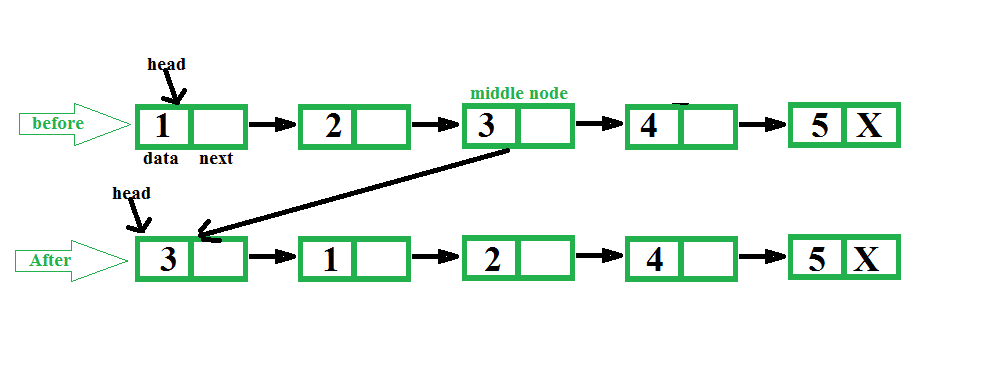GFG App
Open AppBrowser
Continue

# Java Program For Making Middle Node Head In A Linked List

Given a singly linked list, find middle of the linked list and set middle node of the linked list at beginning of the linked list.
Examples:

```Input: 1 2 3 4 5
Output: 3 1 2 4 5

Input: 1 2 3 4 5 6
Output: 4 1 2 3 5 6 ```The idea is to first find middle of a linked list using two pointers, first one moves one at a time and second one moves two at a time. When second pointer reaches end, first reaches middle. We also keep track of previous of first pointer so that we can remove middle node from its current position and can make it head.

## Java

 `// Java program to make middle node ` `// as head of Linked list` `public` `class` `GFG ` `{     ` `    ``// Link list node ` `    ``static` `class` `Node ` `    ``{` `        ``int` `data;` `        ``Node next;` `        ``Node(``int` `data)` `        ``{` `            ``this``.data = data;` `            ``next = ``null``;` `        ``}` `    ``}` `    `  `    ``static` `Node head;` `    `  `    ``/* Function to get the middle and ` `       ``set at beginning of the linked list*/` `    ``static` `void` `setMiddleHead()` `    ``{` `        ``if` `(head == ``null``)` `            ``return``;` `     `  `        ``// To traverse list nodes one ` `        ``// by one` `        ``Node one_node = head;` `     `  `        ``// To traverse list nodes by ` `        ``// skipping one.` `        ``Node two_node = head;` `     `  `        ``// To keep track of previous of middle` `        ``Node prev = ``null``;` `        ``while` `(two_node != ``null` `&& ` `               ``two_node.next != ``null``) ` `        ``{     ` `            ``// For previous node of middle node ` `            ``prev = one_node;` `     `  `            ``// Move one node each time` `            ``two_node = two_node.next.next;` `     `  `            ``// Move two node each time` `            ``one_node = one_node.next;` `        ``}` `     `  `        ``// Set middle node at head ` `        ``prev.next = prev.next.next;` `        ``one_node.next = head;` `        ``head = one_node;` `    ``}` `     `  `    ``// To insert a node at the beginning ` `    ``// of linked list.` `    ``static` `void` `push(``int` `new_data)` `    ``{` `        ``// Allocate node ` `        ``Node new_node = ``new` `Node(new_data);` `     `  `        ``// Link the old list of the new node ` `        ``new_node.next = head;` `     `  `        ``// Move the head to point to the ` `        ``// new node ` `        ``head = new_node;` `    ``}` `     `  `    ``// A  function to print a given linked list` `    ``static` `void` `printList(Node ptr)` `    ``{` `        ``while` `(ptr != ``null``) ` `        ``{` `            ``System.out.print(ptr.data + ``" "``);` `            ``ptr = ptr.next;` `        ``}` `        ``System.out.println();` `    ``}` `     `  `    ``// Driver function` `    ``public` `static` `void` `main(String args[])` `    ``{` `        ``// Create a list of 5 nodes` `        ``head = ``null``;` `        ``int` `i;` `        ``for` `(i = ``5``; i > ``0``; i--)` `            ``push(i);` `        `  `        ``System.out.print(``" list before: "``);` `        ``printList(head);` `     `  `        ``setMiddleHead();` `     `  `        ``System.out.print(``" list After:  "``);` `        ``printList(head);` `    `  `    ``}` `}` `// This code is contributed by Sumit Ghosh`

Output:

```list before: 1 2 3 4 5
list After : 3 1 2 4 5 ```

Time Complexity: O(n) where n is the total number of nodes in the linked list.

Space Complexity: O(1) since using constant space